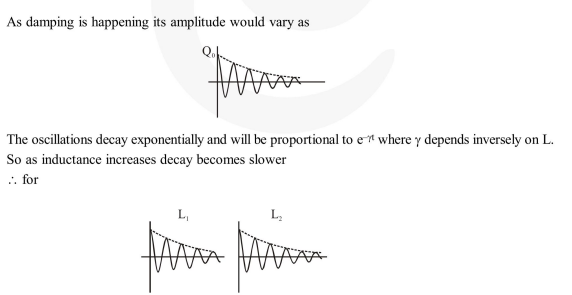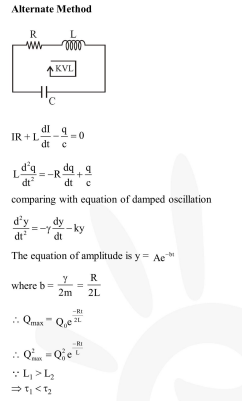# An LCR circuit is equivalent to a damped pendulum.

Question:

An LCR circuit is equivalent to a damped pendulum. In an LCR circuit the capacitor is charged to $\mathrm{Q}_{0}$ and then connected to the $\mathrm{L}$ and $\mathrm{R}$ as shown below. If a student plots graphs of the square of maximum charge $\left(\mathrm{Q}_{\text {Max }}^{2}\right)$ on the capacitor with time $(\mathrm{t})$ for two different values $\mathrm{L}_{1}$ and $\mathrm{L}_{2}\left(\mathrm{~L}_{1}>\mathrm{L}_{2}\right)$ of $L$ then which of the following represents this graph correctly ? (plots are schematic and not drawn to scale)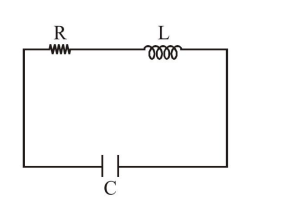1.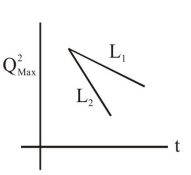2.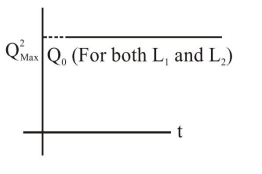3.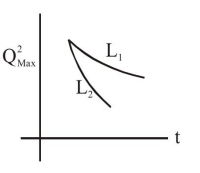4.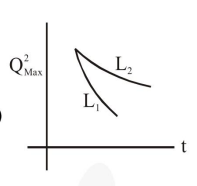Correct Option: , 3

Solution: